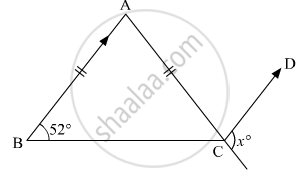# In the Given Figure, Abc is an Isosceles Triangle Whose Side Ac is Produced to E. Through C, Cd is Drawn Parallel to Ba. the Value of X is - Mathematics

MCQ

In the given figure, ABC is an isosceles triangle whose side AC is produced to E. Through C, CD is drawn parallel to BA. The value of x is• 52°

• 76°

• 156°

• 104°

#### Solution

We are given that;

ΔABC , is isosceles

AB = AC

∠B = ∠C

∠C = 52

And  AB || CD

We are asked to find angle xFrom the figure we have

∠ACB = 52°

Therefore,

∠A = 180° - 2 xx 52°

= 76°

Since AB || DC , so

∠ACD = ∠BAC

= 76°

Now

x + 76 = 180

= 180 - 76

= 104

Concept: Criteria for Congruence of Triangles
Is there an error in this question or solution?

#### APPEARS IN

RD Sharma Mathematics for Class 9
Chapter 12 Congruent Triangles
Exercise 12.8 | Q 15 | Page 87
Share